# Ideal gas and Laws

Ideal gas :- it is that gas which obeys the gas laws and obey ideal gas equation.

Characteristics of ideal gas :-

1. The size of the molecule of a gas is zero.
2. There is no force of attraction or repulsion among the molecules of the gas.
• There is no ideal gas; however at low press use & high temperature, some real gas like H2, O2, He etc obeys the ideal gas behavior.
• At low pressure & high gas obeys the relation :-

PV = KT

& K is a constant i.e.

 K= NKB

N number of molecules of the gas  KB botzmann constant = 1.38 x 10-23 J/K         So

 PV = NKB

For two gases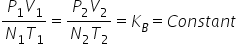So if P,V,T are same then N is same for all the gases.

Avagadro’s Hypothesis :- equal volume of all gases contains equal number of molecules under same temperature and pressure.

NA => Avagadro’s number = 6.02 x 1023

Use of Avagardro’s number

1. To calculate number of atom :- No of atoms in m gram =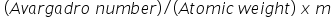2. To calculate number of molecules :- Number of molecules =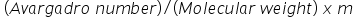3. To calculate number of  molecules in a given Volume of gas :- =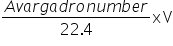4.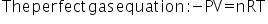5. R = 8.314 J mol-1   or R = 0.0821 atm L mol-1 K-1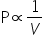( at constant temp ) or PV = constant

Charle’s law :- for a given mass of a gas; volume of a gas is directly proportional to temperature at constant pressure.

P α V ( at constant temperature.)

Dalton’s law of partial pressure :-  according to this law when two or more than two non-reacting gases combine with each other than the total pressure is given by the sum of the partial pressure of the gases.

P = P1 + P2 + P3.

Postulates of KINETIC THEORY OF GAS :-

1. Gases are made up of large number of particles called molecules which are vey small in size.
2. All gas molecules have same mass and they are in random motion.
3. Volume occupied by the gas molecules is negligible as compared to total volume occupied by the gas.
4. There is no inter molecular force of attraction between the gas molecules.
5. There is a collision between the gas molecules & the collision is elastic.
6. Pressure exerted by a gas is due to bombardment of gas molecules on the wall of container.
7. The kinetic energy of gas molecules is directly proportional to the temperature.
Related Keywords